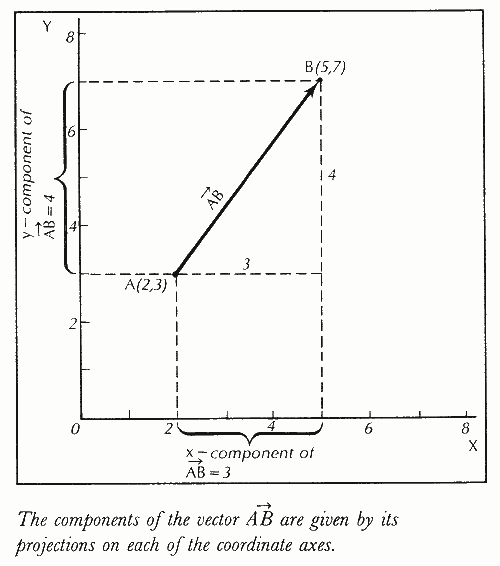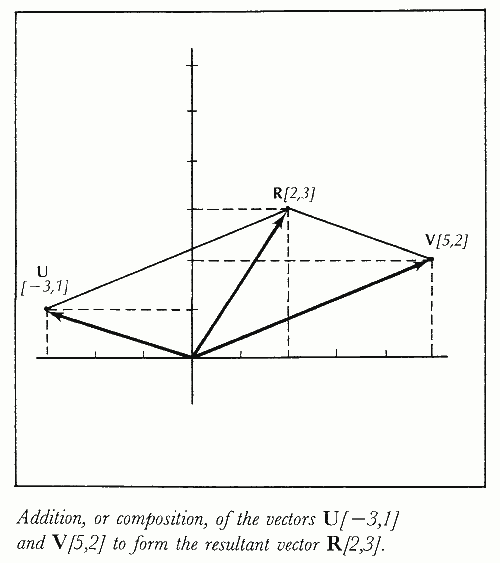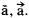# vector

(redirected from Vectorizing)
Also found in: Dictionary, Thesaurus, Medical.

## vector,

quantity having both magnitude and direction; it may be represented by a directed line segment. Many physical quantities are vectors, e.g., force, velocity, and momentum. Thus, in specifying a force, one must state not only how large it is but also in what direction it acts.

### Representation and Reference Systems

The simplest representation of a vector is as an arrow connecting two points. Thus,is used to designate the vector represented by an arrow from point A to point B, whiledesignates a vector of equal magnitude in the opposite direction, from B to A. In order to compare vectors and to operate on them mathematically, however, it is necessary to have some reference system that determines scale and direction. Cartesian coordinatesCartesian coordinates
[for René Descartes], system for representing the relative positions of points in a plane or in space. In a plane, the point P is specified by the pair of numbers (x,y
are often used for this purpose. In the plane, two axes and unit lengths along each axis serve to determine magnitude and direction throughout the plane. For example, if the point A mentioned above has coordinates (2,3) and the point B coordinates (5,7), the size and position of the vector are thus determined. The size of the vector in the x-direction is found by projecting the vector onto the x-axis, i.e., by dropping perpendicular line segments to the x-axis. The length of this projection is simply the difference between the x-coordinates of the two points A and B, or 5 − 2 = 3. This is called the x-component of the vector. Similarly, the y-component of the vector is found to be 7 − 3 = 4. A vector is frequently expressed by giving its components with respect to the coordinate axes; thus, our vector becomes [3,4].Knowledge of the components of a vector enables one to compute its magnitude—in this case, 5, from the Pythagorean theorem [(32 + 42)1/2 = 5)]—and its direction from trigonometry, once the lengths of the sides of the right triangle formed by the vector and its components are known. (Trigonometry can also be used to find the component of the vector as projected in some direction other than the x-axis or y-axis.) Since the vector points from A to B, both its components are positive; if it pointed from B to A, its components would be [−3,−4] but its magnitude and orientation would be the same.

It is obvious that an infinite number of vectors can have the same components [3,4], since there are an infinite number of pairs of points in the plane with x- and y-coordinates whose respective differences are 3 and 4. All these vectors have the same magnitude and direction, being parallel to one another, and are considered equal. Thus, any vector with components a and b can be considered as equal to the vector [a,b] directed from the origin (0,0) to the point (a,b). The concept of a vector can be extended to three or more dimensions.

### Addition and Multiplication of Vectors

The addition, or composition, of two vectors can be accomplished either algebraically or graphically. For example, to add the two vectors U [−3,1] and V [5,2], one can add their corresponding components to find the resultant vector R [2,3], or one can graph U and V on a set of coordinate axes and complete the parallelogram formed with U and V as adjacent sides to obtain R as the diagonal from the common vertex of U and V.Two different kinds of multiplication are defined for vectors in three dimensions. The scalar, or dot, product of two vectors, A and B, is a scalar, or quantity that has a magnitude but no direction, rather than a vector, and is equal to the product of the magnitudes of A and B and the cosine of the angle θ between them, or AB = |A| |B| cos θ. The vector, or cross, product of A and B is a vector, A × B, whose magnitude is equal to |A| |B| sin θ and whose orientation is perpendicular to both A and B and pointing in the direction in which a right-hand screw would advance if turned from A to B through the angle θ. The vector product is an example of a kind of multiplication that does not follow the commutative lawcommutative law,
in mathematics, law holding that for a given binary operation (combining two quantities) the order of the quantities is arbitrary; e.g., in addition, the numbers 2 and 5 can be combined as 2+5=7 or as 5+2=7.
, since A × B = −B × A.

### Vector Analysis and Vector Space

The components of a vector need not be constants but can also be variables and functionsfunction,
in mathematics, a relation f that assigns to each member x of some set X a corresponding member y of some set Y; y is said to be a function of x, usually denoted f(x) (read "f of x ").
of variables. For example, the position of a body moving through space can be described by a vector whose x, y, and z components are each functions of time. The methods of the calculuscalculus,
branch of mathematics that studies continuously changing quantities. The calculus is characterized by the use of infinite processes, involving passage to a limit—the notion of tending toward, or approaching, an ultimate value.
may be applied to such vector functions, leading to the branch of mathematics known as vector analysis.

The more general extension of vectors leads to the concept of a vector space. A vector space is a set of elements, A, B, C, … , called vectors, for which the operations of addition of vectors and multiplication of a vector by a scalar are defined and which satisfies ten axioms relating to such properties as closure under both operations, associativity, commutativity, and existence of a zero vector, an additive inverse (negative of a vector), and a unit scalar.

### Bibliography

See P. Gustyatnikov and S. Reznichenko, Vector Algebra (1988); J. E. Marsden and A. Tromba, Vector Calculus (1988).

## Vector

in the geometric sense, a directed line segment—that is, a segment whose beginning (also called the point of application) and end are indicated. Vectors are denoted by bold Latin letters a, b, … or the ordinary letters with lines or arrows above them:A vector that starts at point A and ends at point B is denoted by. The straight line on which the vector is located is called the line of action of the given vector.

The concept of a vector arose in connection with the study of quantities that were characterized by a numerical value and a direction—for example, the displacement, velocity, and acceleration of a moving material point; the force acting on it; and so forth. Mechanics and physics classify vectors as free, sliding, or bound. A free vector is a vector whose value is not changed by an arbitrary, parallel displacement. An example of a free vector is the velocity of motion of a material point. A vector is called sliding if its value is not changed by any parallel displacement along its line of action. An example of a sliding vector is a force acting on an absolutely rigid body (two forces that are equal and located on the same straight line produce identical actions on an absolutely rigid body). A bound vector has its point of application fixed. For example, a force applied to a certain point of an elastic body is a bound vector. The properties of free vectors are studied in vector algebra. The general concept of a vector as an element of a so-called vector space is defined axiomatically.

### REFERENCE

Il’in, V. A., and E. G. Pozniak. Analiticheskaia geometriia. Moscow, 1968.

E. G. POZNIAK

## Vector

(in medicine), an arthropod that transfers the causative agent of an infectious or parasitic disease between humans and animals. Insects that can act as vectors include such bloodsuckers as fleas, true lice, mosquitoes, members of the family Phlebotomidae, simuliids, and tabanids. Ticks, mites, and non-bloodsucking insects can also be vectors, for example, flies, cockroaches, and ants. A distinction is made between a biological vector, in whose body the causative agent develops and reproduces, and a mechanical vector, which is not essential to the causative agent’s life cycle. For example, the malarial plasmodium develops inside a mosquito that acts as a biological vector, while the causative agents of intestinal infections are transferred by mechanical vectors.

Bloodsucking vectors transmit a causative agent while they suck blood. For instance, malaria and yellow fever are transmitted by mosquitoes, tick-borne encephalitis by ticks, and plague by fleas. The causative agent of an infection may penetrate the human body when the excrement of bloodsucking insects falls on injured skin, as in the transmission of European typhus by lice, or when the blood of crushed vectors falls on injured skin, as in relapsing fever. To transmit an infection, a bloodsucking vector must first suck the blood of an affected human or animal, which act as sources of infection. In certain diseases, for example, malaria and European typhus, only humans can be sources, while in tick-borne encephalitides, relapsing fever, phlebotomus fever, and leishmaniases, such animals as rodents, wolves, birds, dogs, cows, and goats can be sources.

The diseases that are carried by bloodsucking vectors are called transmissible diseases. Several vectors, for example, the ticks that transfer the virus of tick-borne encephalitis, transmit viruses to their own offspring from generation to generation. A natural geographic focus of infection can arise where a reservoir of infection exists among wild animals and where the causative agents can circulate among those animals and the vectors. Non-bloodsucking insects carry causative agents on their legs and hairs and in their excrement.

Vectors are controlled by insecticides. Repellents, mosquito netting, and overalls worn during travel in areas that are infested with ticks and mites protect against bites.

V. L. VASILEVSKII

## vector

[′vek·tər]
(computer science)
(mathematics)
An element of a vector space.
A matrix consisting of a single row or a single column of entries.
(medicine)
An agent, such as an insect, capable of mechanically or biologically transferring a pathogen from one organism to another.
To guide a pilot, navigator, aircraft, or missile from one point to another within a given time by means of a direction communicated to the craft.
(physics)
A quantity which has both magnitude and direction, and whose components transform from one coordinate system to another in the same manner as the components of a displacement. Also known as polar vector.

## vector

i. A quantity having both magnitude and direction. In air navigation, it is normally expressed graphically as, as in expressing wind speed and direction. The first three digits indicate direction, and the next two or three digits after the stroke indicate the wind speed.
ii. To guide a pilot, navigator, aircraft, or missile from one point to another within a given time by means of a vector communicated to the craft, as in “He vectored the fighter to the intruder.” To instruct a pilot on which heading to take, as in “He vectored him to the alternate airfield.”

## vector

1. Maths a variable quantity, such as force, that has magnitude and direction and can be resolved into components that are odd functions of the coordinates. It is represented in print by a bold italic symbol: F
2. Maths an element of a vector space
3. Pathol an organism, esp an insect, that carries a disease-producing microorganism from one host to another, either within or on the surface of its body
4. Genetics an agent, such as a bacteriophage or a plasmid, by means of which a fragment of foreign DNA is inserted into a host cell to produce a gene clone in genetic engineering
5. the course or compass direction of an aircraft

## vector

(mathematics)
A member of a vector space.

## vector

(graphics)
A line or movement defined by its end points, or by the current position and one other point. See vector graphics.

## vector

(operating system)
A memory location containing the address of some code, often some kind of exception handler or other operating system service. By changing the vector to point to a different piece of code it is possible to modify the behaviour of the operating system.

Compare hook.

## vector

(programming)
A one-dimensional array.

## vector

(1) In computer graphics, a line designated by its end points (X-Y or X-Y-Z coordinates). When a circle is drawn, it is made up of many small vectors. See vector graphics and graphics.

(2) In matrix algebra, a one-row or one-column matrix.

(3) An airplane's travel path.

(4) The term is used generically for "pathway" or "avenue" or even an influence of some type. For example, "x, y and z provide multiple threat vectors for malware intrusion" means there are multiple ways for intrusion to occur.
References in periodicals archive ?
2 Vectorizing Compiler:Development software used to build optimized programs for vector supercomputers.
There is only a minor profit when vectorizing the longest loop instead of the i-loop, which uses vector length 1 for some levels.
Anyway, if your workstation spends less than 80% of its time executing the inner loops of your code, then it is very unlikely to be sped up appreciably by a vectorizing compiler.
A major component in the success of vector supercomputers is the ability of scientists to write Fortran programs in a "vectorizable" style and expect vectorizing compilers to automatically produce efficient code [8, 35].
This strongly suggests that either (1) the conventional restructuring capabilities of our prototype sparse compiler performing ordinary program transformations should be improved or (2) programmers using the sparse compiler should adhere to a few rules about how the original program should look like (as has been common practice in using early vectorizing compilers).
Conservative dependence analysis methods may be efficacious for the demands of vectorizing compilers.
As in vectorizing and parallelizing compilers, dependence is a critical compiler tool for performing transformations to improve the memory performance of loops.
Furthermore, one could perhaps add a simple vectorizing siever to each one along with some special hardware that would make finding the roots of the polynomial easier.

Site: Follow: Share:
Open / Close